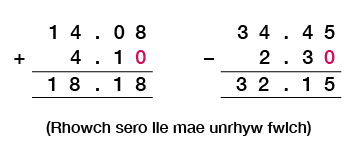Science, Maths & Technology

### Become an OU studentEveryday maths 1 (Wales)

Start this free course now. Just create an account and sign in. Enrol and complete the course for a free statement of participation or digital badge if available.

# 4.3 Calculations using decimals

When you make any calculation with decimals – that is, addition, subtraction, multiplication and division – it is very important to make sure that the decimal point is in the correct place. If you don’t, you’ll get the wrong answer.

When we add or subtract decimals, it is important to line up the decimal points.

Calculate the following:

1. 14.08 + 4.1
2. 34.45 – 2.3

### MethodFigure 24 Calculating using decimals

Now try the following activity. Remember to check your answers once you have completed the questions.

## Activity 27: Using decimals

Now try the following activity using written methods. Remember to check your answers once you have completed the questions.

1. 4.2 + 3.7
2. 6.7 – 5.1
3. 42.19 + 13.5
4. 74.8 – 24.3
5. £163.25 + £27.12
6. 2.1 m – 0.75 m